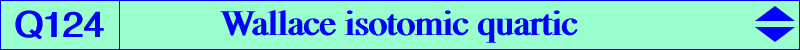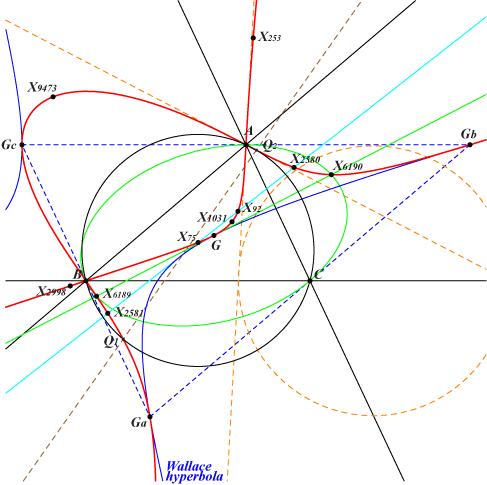∑ (b^2 – c^2) y^2 z^2 = 0X(2), X(75), X(92), X(253), X(1031), X(2580), X(2581), X(2998), X(6189), X(6190), X(9473), X(19712), X(19713), X(19776), X(19777), X(24243), X(24244), X(34287), X(35058), X(39699), X(39705), X(39718), X(39727), X(39733), X(39735), X(40042), X(46275) vertices of the antimedial triangle more generally, vertices of the anticevian triangle of any of its points Q1, Q2 : points on (O) and the line X(22)X(157)Q124 is a quartic very similar to Q066 where a generalization is given. In fact, the barycentric product of Q124 by X(1) is Q066. Q124 is the isotomic transform of the Wallace diagonal rectangular hyperbola which is the anticomplement of the Kiepert hyperbola. It contains X(1), X(2), X(20), X(63), X(147), X(194), X(487), X(488), X(616), X(617), X(627), X(628), X(1670), X(1671), X(1764), X(2128), X(2582), X(2583), X(2896), etc. Q124 is also the square root of the Kiepert hyperbola. In other words, Q124 contains the square roots of all the points on the Kiepert hyperbola. Q124 is the locus of M whose cevian circle passes through X(125), together with the Jerabek hyperbola. Hence, Q124 is also the cyclocevian transform of the Jerabek hyperbola i.e. the cyclocevian conjugate M' of a point M on the Jerabek hyperbola ends up on Q124. The points A, B, C are nodes. The nodal tangents pass through the traces of the circles orthogonal to the circum-circle and centered at the intersections of the perpendiculars at A, B, C to the Euler line with the sidelines of ABC. See also the Wallace isogonal quartic Q182.